# Inclined Plane

\$150.58

Code Number: P0101012_Inclined Plane

Brand: AELAB

#### Purpose:

1. Measure the component Fpp of the weight of an object which parallel to the inclined plane as a function of the angle of inclination α. Comparing to Theory

2. Plot the ratio of the component Fpp to the weight G as a function of sin α

3. Calculate the component of force normal to the plane Fv.

- +

#### Inclined plane experiment including:

1. Inclined plane apparatus:

- Attaching angle dial: 0 - 90 degrees

- Tilt range: 0 - 40 degrees

- Pulley attaching mechanisium. Height Adjustable.

2. Precision Pulley

3. Wooden Wedge

4. Roller: Mass: 410g, aluminium, and mass: 1060g, steel,  with hook.

5. Weight: 12 x 50g, 10 x 10g, 1 x 5g, và 2 x 2g.

1. Measure the component Fpp of the weight of an object which parallel to the inclined plane as a function of the angle of inclination α. Comparing to Theory

2. Plot the ratio of the component Fpp to the weight G as a function of sin α

3. Calculate the component of force normal to the plane Fv.

# THEORY AND PRACTICE

The inclined plane exercise is not difficult for any student because it is easy and familiar.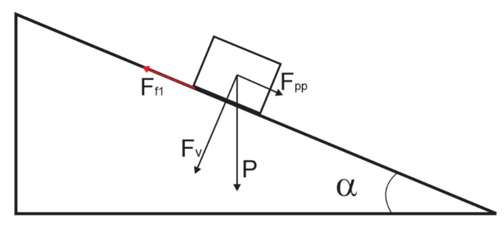An object m lying on an inclined plane. It is affected by gravity P and friction force Ff1.

Gravity P is analyzed into 2 components:

+ Components lying along the inclined plane: FPP = P.sin (α)

+ Components perpendicular to the inclined plane: FV = P.cos (α)

P = FPP + FV (Vector)

Friction force: Ff1 = Fv.μ  (μ: Friction coefficient)

By experimentally, measuring the force component lying parallel to the inclined plane. And comparing to theory.

## Method 1: Measure with dynamometer.

Hook the dynamometer to the object to be measured as shown below: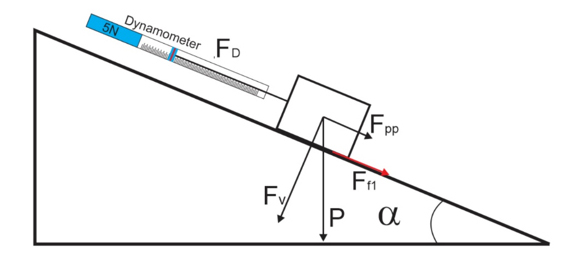Now the measured value of the dynamometer FD is the sum of the gravity components parallel to the plane Fpp and the friction force between the object and the plane Ff1.

And there is no way to measure the Fpp component.

This way is not suitable.

## Method 2: Use weights.

Installation:

We install the experiment as shown below: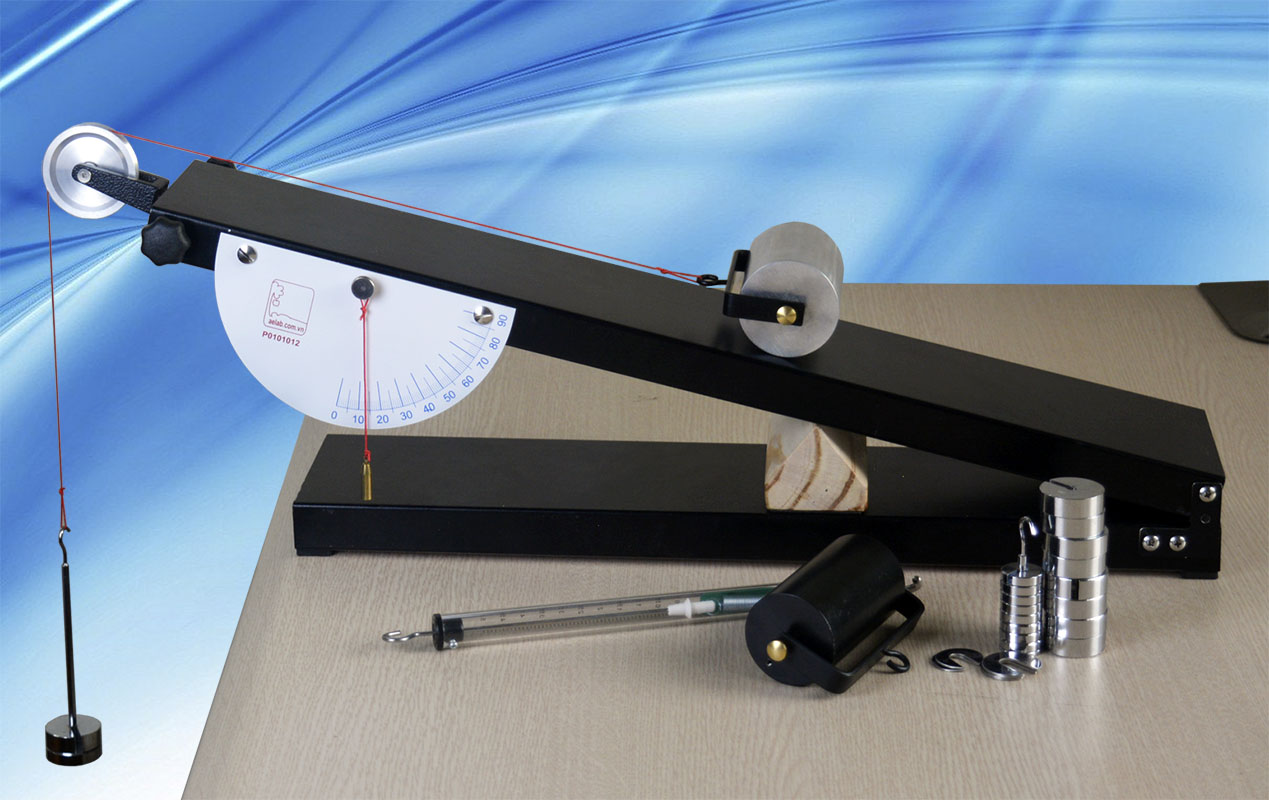Inclined plane apparatus

Adjust so that the connecting cord is parallel to the inclined plane: By adjusting the pulley height## Procedure:

Adjust the wood wedge so that the plane is tilted at an angle of 10, 15, 20, 25, 30, 35 degrees.

Add/remove weights so that the roller roll very slowly up onto the inclined plane.

When the roller moves slowly upwards, it is assumed that the acceleration is zero.#### Balance the force.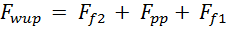(1)

We add/remove the weights so that the roller moves very slowly downwards.

When the roller moves slowly downwards.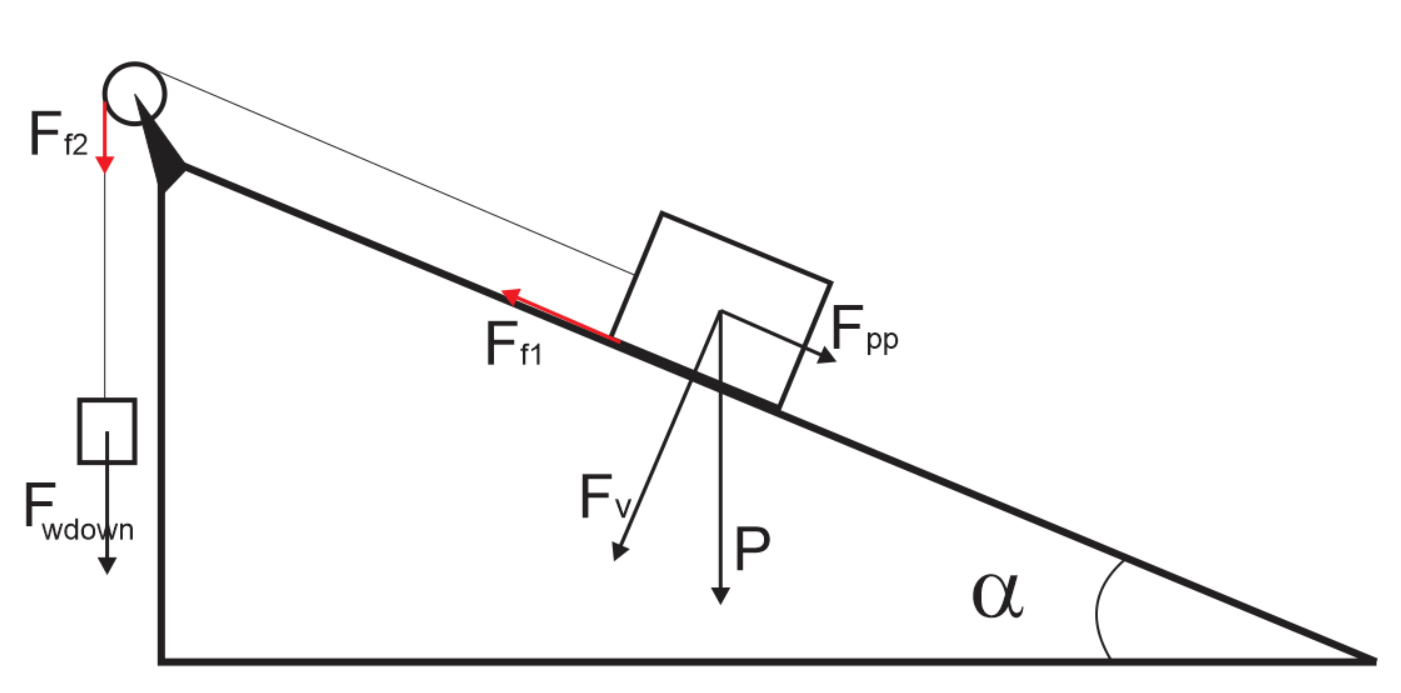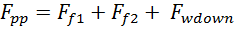(2)

From (1) and (2):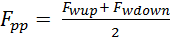(3)

In Theory: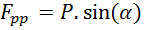(4)

## Experimental results:

We do the experiment with the roller m = 1060g, P = 10.60N

We work with the angles: 10, 15, 20, 25, 30, 35 degrees.

Make the table as follows: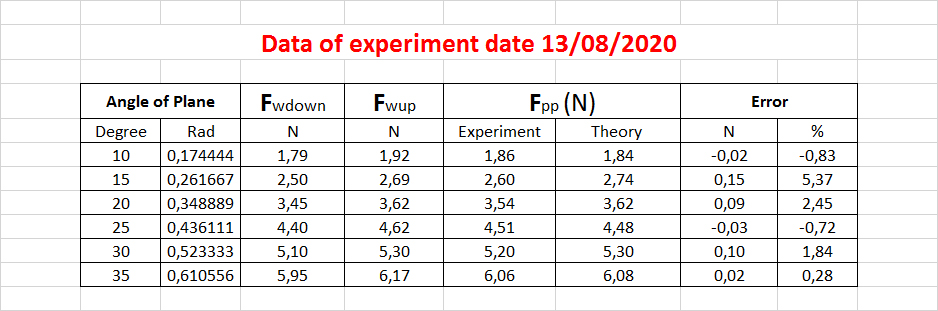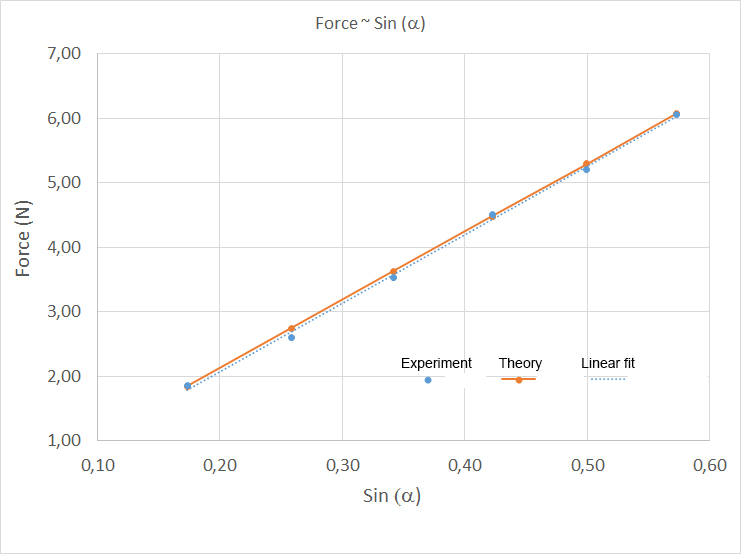Inclined plane apparatus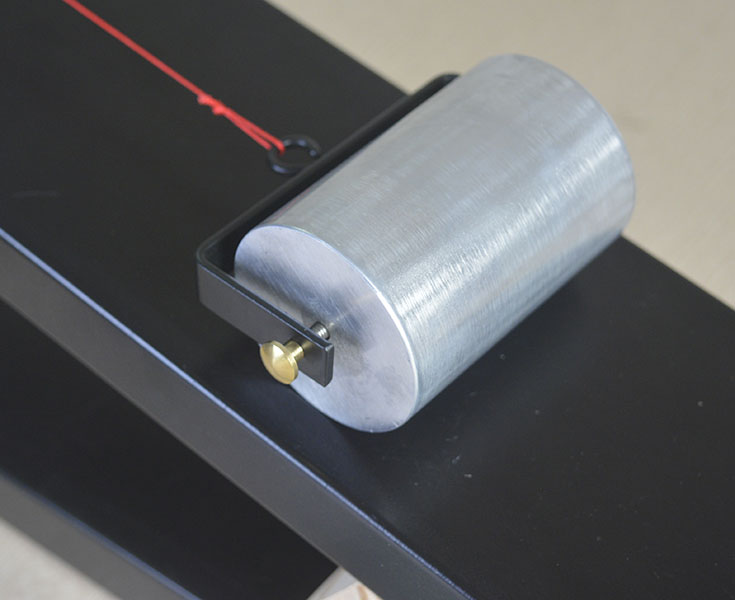Inclined plane apparatus: Aluminium Roller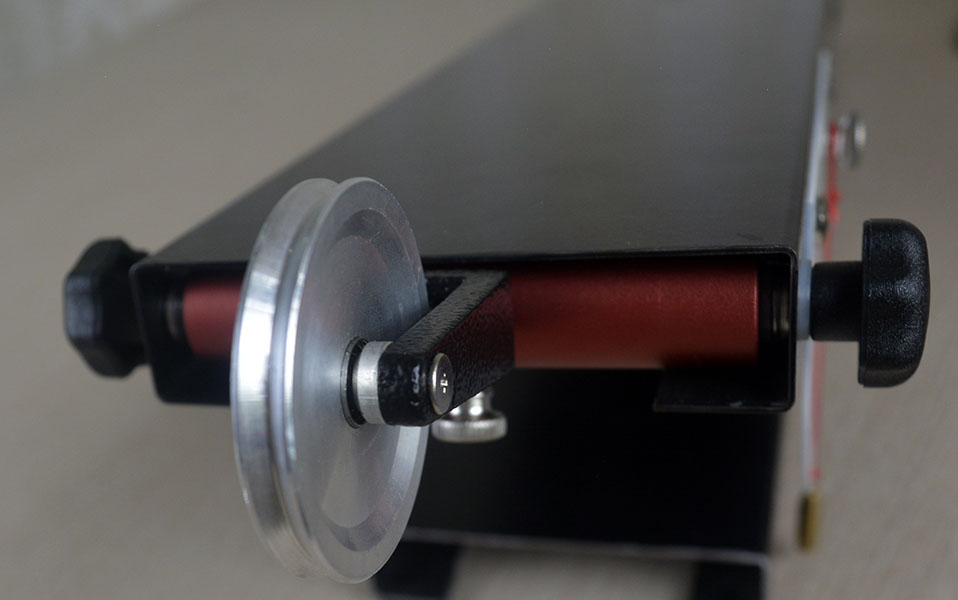Inclined plane apparatus: Pulley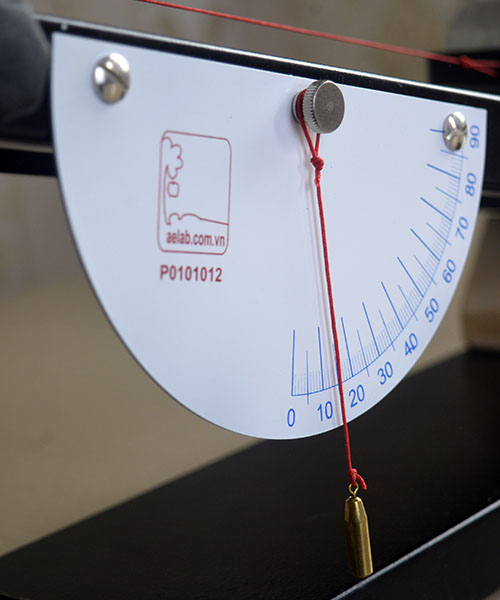Inclined plane apparatus: Angle scale